U.S. Department of Transportation
1200 New Jersey Avenue, SE
Washington, DC 20590
202-366-4000

Federal Highway Administration Research and Technology
Coordinating, Developing, and Delivering Highway Transportation InnovationsThis report is an archived publication and may contain dated technical, contact, and link information
 Federal Highway Administration > Publications > Research Publications > 98133 > Ch06_03.Cfm > Accident Models for Two-Lane Rural Roads: Segment and Intersections
Publication Number: FHWA-RD-98-133
Date: October 1998

# Accident Models for Two-Lane Rural Roads: Segment and Intersections

## 6. Validation and Further Analysis

Cumulative Scaled Residuals

Figures 8 through 15 below show cumulative scaled residual plots for the extended negative binomial model (combined segments, Table 27) and for negative binomial models (Minnesota three-legged and four-legged intersections, Table 35). The cumulative scaled residuals are plotted against leading explanatory variables. For an explanatory variable x, a plot is made of j versus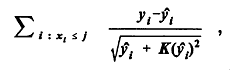where j runs through the values of x. Each term, a scaled residual, should be approximately unbiased. However, if the sum depends in some regular way on j, then the model may have missed some systematic effects (e.g., quadratic dependency). If there is no systematic effect and the terms are otherwise independent, the expected value of the sum is approximately zero, and its standard deviation is approximately the square root of the number of observations for which x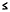j. For the segments this means a standard deviation not in excess of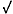1331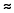36.5 and for the intersections one not in excess of38919.7 (three-legged) or32718.1 (four-legged). The cumulative scaled residuals should represent the net distance traveled after each step in a random walk that ends at the sum of the scaled residuals for the entire data set.

For the segments (Figures 8, 9, 10, and 11) the overall sum of the scaled residuals is about -8, for the three-legged intersections (Figures 12 and 13) the sum is about -2, and for the four-legged intersections (Figures 14 and 15) the sum is about +1. Thus the segment graphs and the three-legged graphs should end below the horizontal axis, while the four-legged graphs should end above.

Table 48 summarizes the residual behavior.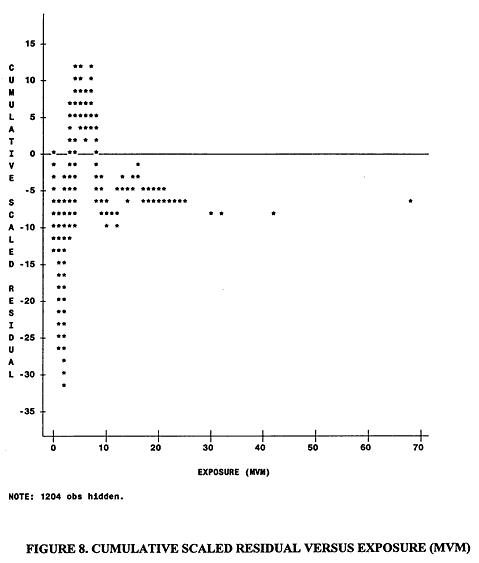The segment model overpredicts (predicted mean number of accidents higher than actual number) at the low end of exposure. The cumulative scaled residual varies from -32 to +12.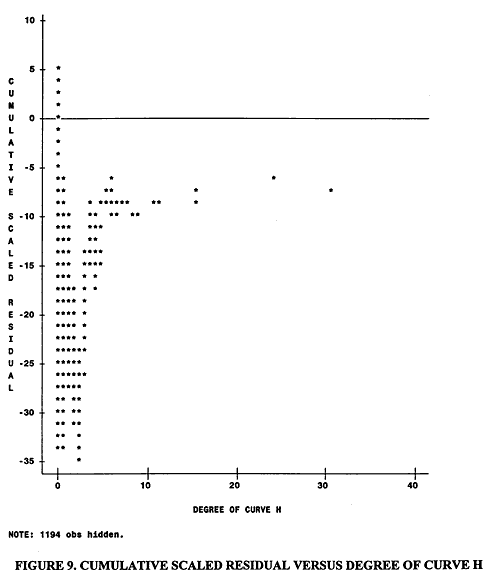Overprediction occurs on segments without horizontal curves. The cumulative scaled residual varies from -36 to +7.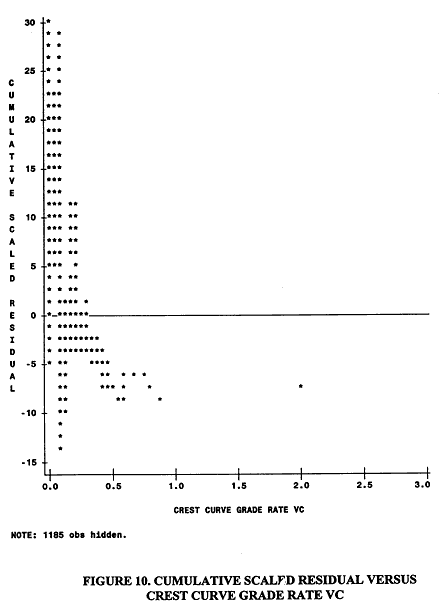The segment model underpredicts on segments without crest curves. The cumulative scaled residual varies from -13 to +30 .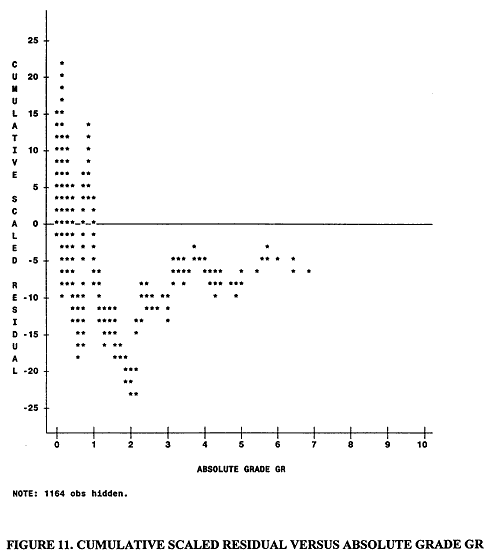The cumulative scaled residual varies from -24 to + 22.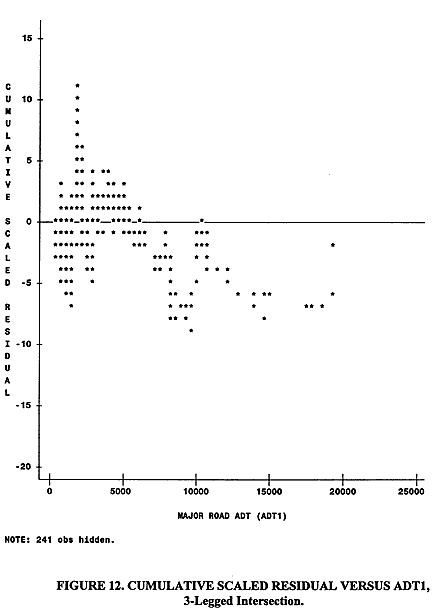The cumulative scaled residual varies from -9 to +11.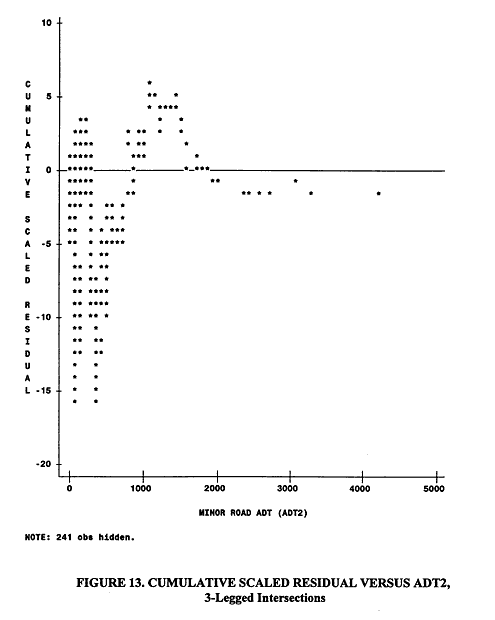The cumulative scaled residual varies from -16 to +7.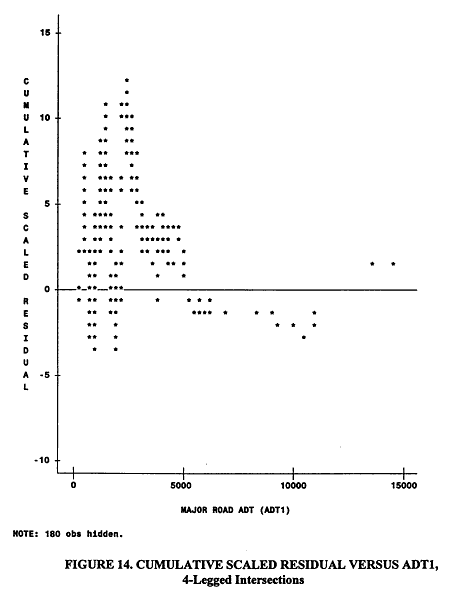The cumulative scaled residual varies from -4 to +12.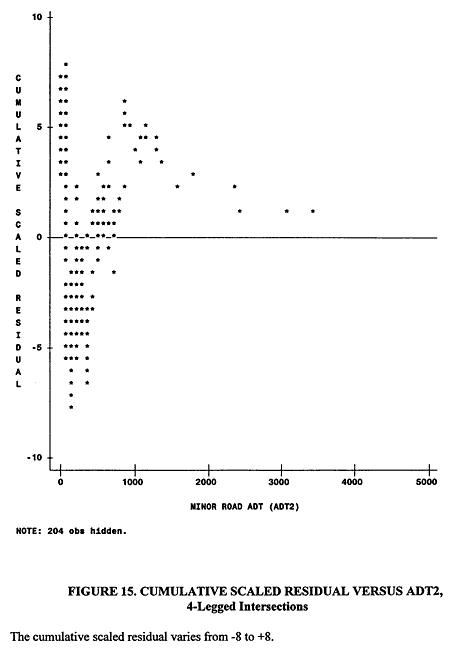.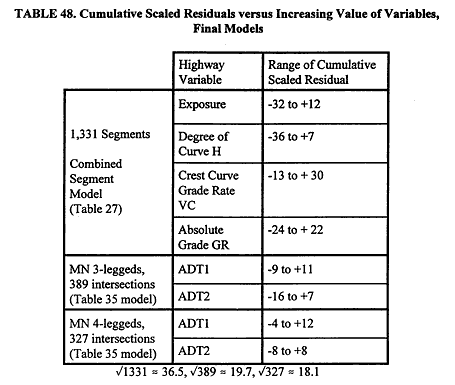Despite the indications of overprediction or underprediction in some regimes in the segment model, which might lead one to develop separate models in different regimes (e.g., one model for low exposure, one for medium exposure, and one for high), the graphs are generally consistent with random walks. In particular the ranges shown in Table 48 above are reasonable. In a random walk, as mentioned, the n-th step or observation on average will take one a distance of less than ±(n)1/2 units from the origin. In addition it is not at all uncommon to stay on one side of zero (above or below) for many steps in succession. Negative binomial models never predict zero values for the dependent variable (in our case numbers of accidents). Thus at low values of highway variables (presumed to be associated with fewer accidents), when the true number of accidents is zero, the negative binomial predicts a positive number and hence must overpredict at least somewhat.